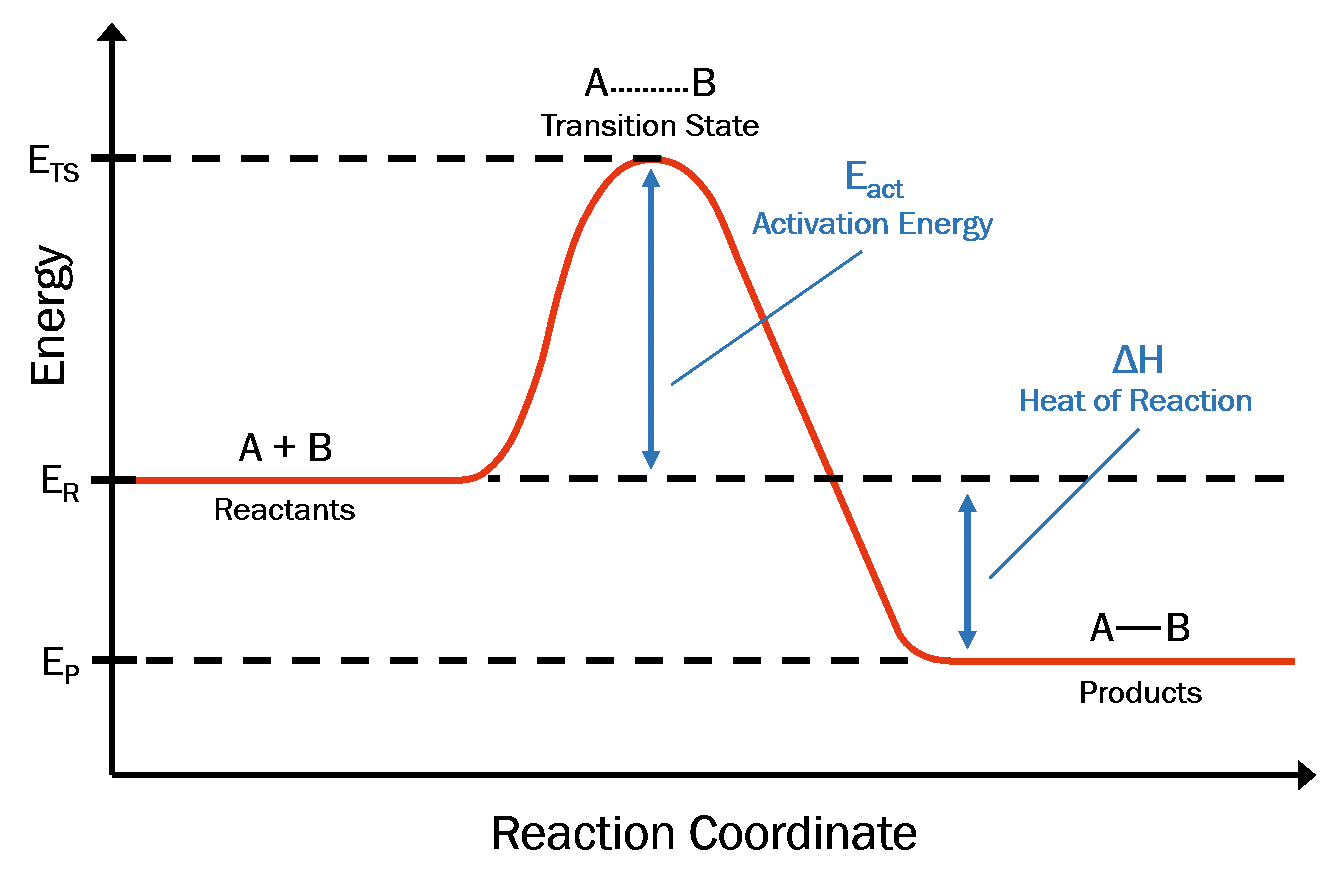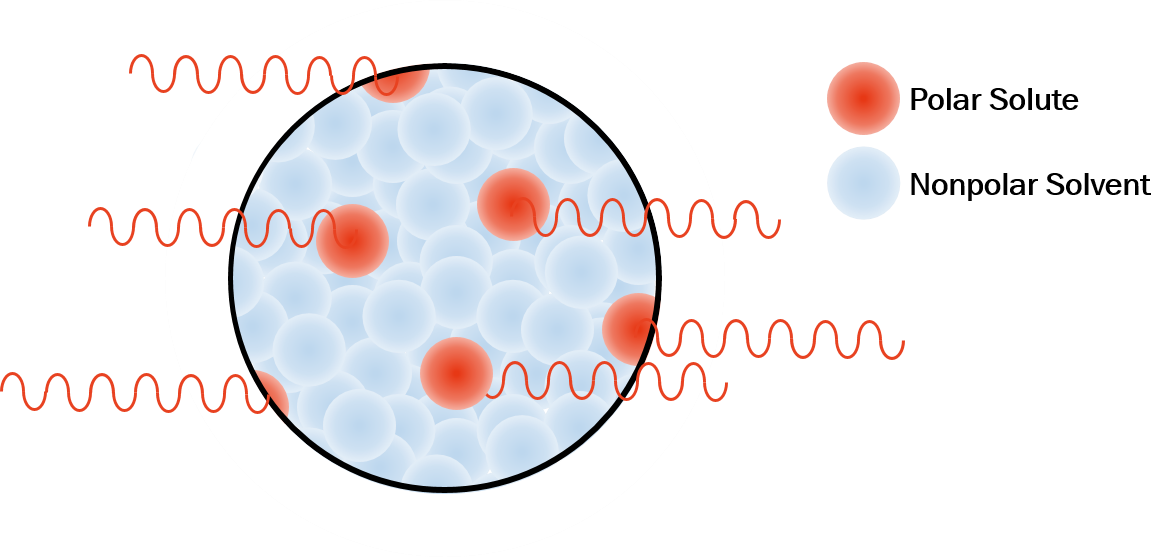Welcome to CEM.com# Microwave Heating - Increasing Reaction Rate

September 23, 2019

Perhaps the largest benefit of microwave heating in chemical synthesis is the dramatic reduction of total synthesis time. Though microwaves are able to heat a solution more efficiently than conventional means (i.e. volumetric heating vs. convective heating), there are other factors that can contribute to the enhanced reaction rates. Let’s take a closer look.

#### How can microwave irradiation increase reaction rate?

To understand how microwaves can impact reaction rate, let’s begin by first reviewing reaction coordinate diagrams. A reaction coordinate diagram plots reaction progress against free energy (Figure 1). In the beginning of a transformation, the reactants are at a particular energy level (ER). In order to react, the reactants must absorb enough energy (i.e. heat) to reach the energy level of their transition state complex (ETS). The difference between ETS and ER is known as the activation energy (EA), and upon overcoming this barrier, reactants undergo rapid product formation (and return to lower energy level, EP).1Figure 1. Reaction coordinate diagram

Next, let’s look at the Arrhenius reaction rate equation (Equation 1). In this equation, reaction rate (k) is dependent on two factors: collision frequency between molecules with proper geometric orientation (A), and the fraction of those molecules that have enough energy to surpass the activation energy barrier (e-Ea/(RT) ). Though microwaves do not influence the pre-exponential factor (A), the activation energy (Ea), or the universal gas constant (R), they can affect the temperature parameter (T); an increase in temperature is met with an increase in reaction rate.1

k = Ae-Ea/(RT)                          (Equation 1)

In most cases, an experiment will perform identically whether heated convectively or through microwave irradiation (assuming all other experimental parameters, including T, are identical); the main benefit of microwave irradiation here being its efficient and volumetric method of heat generation. There are circumstances, however, where microwave irradiation offers dramatic reaction rate improvement.2–5

These circumstances, in particular, occur when a polar (i.e. high microwave-absorbing) solute is present in a nonpolar (i.e. low microwave-absorbing) solvent. Polar reagents absorb microwave energy more readily, resulting in localized molecular superheating above a solution’s bulk temperature. This localized increase in temperature, when factored into the Arrhenius equation, can have a dramatic effect on reaction rate if convective heat transfer to the surrounding medium is slow (Figure 2).2–5Figure 2. Localized superheating of polar solute in a nonpolar solvent

#### How can microwave reactors facilitate reaction rate increase?

In addition to the benefits of direct interactions between matter and microwave irradiation, modern laboratory microwave reactors, such as CEM’s Discover SP, can operate under conditions that further expedite reaction rate. Microwave reactors can perform closed-vessel reactions at temperatures well exceeding reflux while safely maintaining high-pressure atmospheres.

At T = T0 + (10 °C)x, t ≈ (t0)/(2x)                          (Equation 2)

A convenient generalization supported by the Arrhenius equation, is that a reaction rate will approximately double for every 10 °C the temperature increases. With this generalization, the approximation in Equation 2 can be derived, where T = microwave heating temperature, T0 = conventional heating temperature, x = the temperature increase coefficient, t = microwave heating time and t0 = conventional heating time. (For a chart representation of Equation 2, see Table 1). Conventionally heated reactions that once took hours conventionally, can be completed in minutes with laboratory microwave reactors!

Table 1. General microwave reaction rate increase with temperature increase

 Temperature Time T = Conventional 1 h 2 h 3 h 4 h 6 h 8 h 10 h 12 h 16 h 18 h 24 h 48 h 96 h 172 h T + 10 °C 30 min 1 h 1.5 h 2 h 3 h 4 h 5 h 6 h 8 h 9 h 12 h 24 h 48 h 96 h T + 20 °C 15 min 30 min 45 min 1 h 1.5 h 2 h 2.5 h 3 h 4 h 5 h 6 h 12 h 24 h 48 h T + 30 °C 8 min 15 min 23 min 30 min 45 min 1 h 75 min 1.5 h 2 h 2.5 h 3 h 6 h 12 h 24 h T + 40 °C 4 min 8 min 12 min 15 min 23 min 30 min 38 min 45 min 1 h 75 min 1.5 h 3 h 6 h 12 h T + 50 °C 2 min 4 min 6 min 8 min 12 min 15 min 20 min 23 min 30 min 38 min 45 min 1.5 h 3 h 6 h T + 60 °C 1 min 2 min 3 min 4 min 6 min 8 min 10 min 12 min 15 min 20 min 23 min 45 min 1.5 h 3 h T + 70 °C 1 min 2 min 2 min 3 min 4 min 5 min 6 min 8 min 10 min 12 min 23 min 45 min 1.5 h T + 80 °C 1 min 1 min 2 min 2 min 3 min 3 min 4 min 5 min 6 min 12 min 23 min 45 min T + 90 °C 1 min 1 min 2 min 2 min 2 min 3 min 3 min 6 min 12 min 23 min T + 100 °C 1 min 1 min 1 min 2 min 2 min 3 min 6 min 12 min T + 110 °C 1 min 1 min 2 min 3 min 6 min T + 120 °C 1 min 2 min 3 min T + 130 °C 1 min 2 min T + 140 °C 1 min

References

(1)   Brittany, H. (2002). Microwave Synthesis: Chemistry at the Speed of Light. Matthews: CEM Publishing, pp.11-27.